Next: Angular Stability of Floating Up: Hydrostatics Previous: Equilibrium of Floating Bodies

# Vertical Stability of Floating Bodies

Consider a floating body of weightthat, in equilibrium, has a submerged volume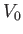. Thus, the body's downward weight is balanced by the upward buoyancy force,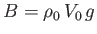: that is,. Let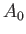be the cross-sectional area of the body at the waterline (i.e., in the plane). It is convenient to define the body's mean draft (or mean submerged depth) as. Suppose that the body is displaced slightly downward, without rotation, such that its mean draft becomes, where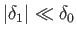. Assuming that the cross-sectional area in the vicinity of the waterline is constant, the new submerged volume is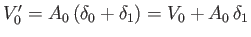, and the new buoyancy force becomes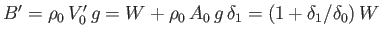. However, the weight of the body is unchanged. Thus, the body's perturbed vertical equation of motion is written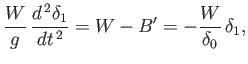(2.19)

which reduces to the simple harmonic equation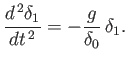(2.20)

We conclude that if a floating body of mean draft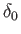is subject to a small vertical displacement then it oscillates about its equilibrium position at the characteristic frequency(2.21)

It follows that such a body is unconditionally stable to small vertical displacements. Incidentally, the previous calculation neglects the phenomenon of added mass, by which some of the water surrounding the floating body oscillates in sympathy with it, thereby increasing the body's effective inertia. (See Sections 5.9 and 7.10.)Next: Angular Stability of Floating Up: Hydrostatics Previous: Equilibrium of Floating Bodies
Richard Fitzpatrick 2016-03-31